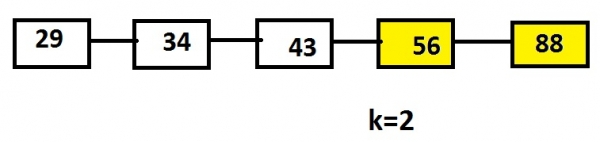# Print the last k nodes of the linked list in reverse order Iterative approach in C language

We have to print the k number of nodes of the linked list in reverse order. We have to apply the iterative approach to solve this problem.

Iterative method is the one which generally uses loops that are executed till the condition holds value 1 or true.

Let’s say, list contains the nodes 29, 34, 43, 56 and 88 and the value of k is 2 than the output will be the alternate nodes till k such as 56 and 88.## Example

Linked List: 29->34->43->56->88
Input: 2
Output: 56 88

Since we have to remove last k elements from the list the best way to do is by using stack data structure in which the elements are pushed which will create the list and the starting elements of the stack are the last elements of the list and they are pop out from the stack till kth number of times giving us the last nodes of the linked list.

The below code shows the c implementation of the algorithm given.

## Algorithm

START
Step 1 -> create node variable of type structure
Declare int data
Declare pointer of type node using *next
Step 2 -> create struct node* intoList(int data)
Create newnode using malloc
Set newnode->data = data
newnode->next = NULL
return newnode
step 3 -> Declare function void rev(struct node* head,int count, int k)
create struct node* temp1 = head
Loop While(temp1 != NULL)
count++
temp1 = temp1->next
end
Declare int array[count], temp2 = count,i
Loop While(temp1 != NULL)
Set array[--temp2] = temp1->data
Set temp1 = temp1->next
End
Loop For i = 0 and i < k and i++
Print array[i]
End
Step 4 -> In Main()
Create list using struct node* head = intoList(9)
Set k=3 and count=0
STOP

## Example

#include<stdio.h>
#include<stdlib.h>
// Structure of a node
struct node {
int data;
struct node *next;
};
//functon for inserting a new node
struct node* intoList(int data) {
struct node* newnode = (struct node*)malloc(sizeof(struct node));
newnode->data = data;
newnode->next = NULL;
return newnode;
}
// Function to reversely printing the elements of a node
void rev(struct node* head,int count, int k) {
while(temp1 != NULL) {
count++;
temp1 = temp1->next;
}
int array[count], temp2 = count,i;
while(temp1 != NULL) {
array[--temp2] = temp1->data;
temp1 = temp1->next;
}
for(i = 0; i < k; i++)
printf("%d ",array[i]);
}
int main() {
printf("reverse of a list is : ");
struct node* head = intoList(9); //inserting elements into a list
}
reverse of a list is : 109 55 24